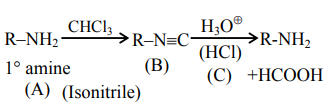# Compound $mathrm{A}$ is converted to B on reaction`
Question:

Compound $\mathrm{A}$ is converted to $\mathrm{B}$ on reaction with $\mathrm{CHCl}_{3}$ and $\mathrm{KOH}$. The compound $\mathrm{B}$ is toxic and can be decomposed by C. A, B and C respectively are :

1. primary amine, nitrile compound, conc. $\mathrm{HCl}$

2. secondary amine, isonitrile compound, conc. $\mathrm{NaOH}$

3. primary amine, isonitrile compound, conc. $\mathrm{HCl}$

4. secondary amine, nitrile compound, conc. $\mathrm{NaOH}$

Correct Option: , 3

Solution: